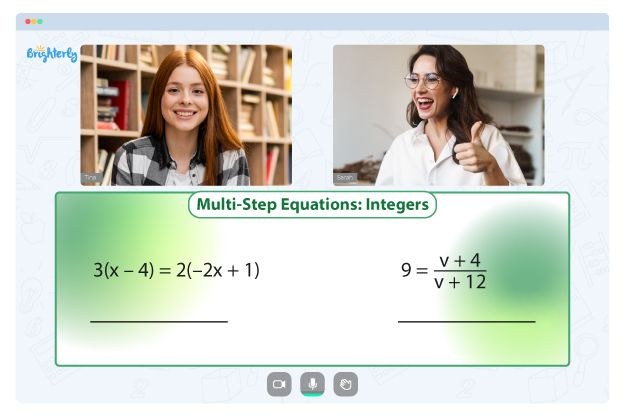# Properties of Exponents Worksheets

Properties of exponents are also called rules of exponents and, in some cases, laws of exponents. These rules work with specific formulas that are used to solve problems. Kids must remember these rules, learn how they work, and master them.

Here are some of the properties of exponents and their formulas for easy recognition:

• Zero exponent rule: a0 = 1
• Identity exponent rule: a1 = a
• Product rule: am × an = am+n
• Quotient rule: am / an = am−n
• Negative exponent rule: a−m = 1/am
• Power of a power rule: (am)n = amn
• Power of a product rule: (ab)m = ambm
• Power of a quotient rule: (a/b)m = am/bm
• Fractional exponents rule:  a1/n = n√a

The exponents’ properties worksheet can help kids learn about the properties of exponents. But, more importantly, these tools can help them understand the properties, one after the other, and apply them in relevant math equations.

## Benefits of exponent properties worksheets

Here are some of the benefits of properties of exponents worksheet answers:

### It makes understanding of properties of exponents easier

The easiest way to get through exponents is by learning all their properties and practicing to use them in equations. Using the properties of exponents worksheet answer key as a learning material allows kids to learn all about the math concept and thus makes it easier for them to work within the exponent’s universe.

### It helps parents understand their children’s strengths and weaknesses

If you are a parent who loves to help their child with studies, then you will find the exponent properties worksheet answers very beneficial. Going through the properties of exponents worksheet with answers together with your kids allows you to understand the exponents and properties of exponents so that you can know how to teach your child effectively.

Math for Kids

Is Your Child Struggling With Math?
1:1 Online Math TutoringYou can find properties of exponents worksheets on internet math websites. Download as many sheets as you can as they are primarily free. They contain a wide range of questions, and kids can easily skip the ones that seem too complex and ask their teachers questions later.### Exponent Properties Worksheet PDF

Exponent Properties Worksheet### Exponent Properties Worksheets PDF

Exponent Properties Worksheets### Properties of Exponents Worksheet Answer Key PDF

Properties of Exponents Worksheet Answer Key### Properties of Exponents Worksheet Answers PDF

Need help with Equations?• Is your child finding it hard to understand equations?
• An online tutor could be of help.

Does your child need extra assistance with grasping of equations? Start learning with an online tutor.